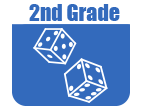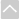Review Activities and Games

These resources are intended for review and practice after initial instruction of the standards.

Strand: MATHEMATICAL PRACTICES (2.MP)Strand: OPERATIONS AND ALGEBRAIC THINKING (2.OA)
Represent and solve problems involving addition and subtraction (Standards 2.OA.1)
Fluently add and subtract within 20 (Standards 2.OA.2).
• Hitting The Target Number
The purpose of this task is to help students develop flexible strategies for adding and subtracting within 20.
• IXL Game: Add and Subtract Numbers
This game helps second graders fluently add and subtract within 20 using mental strategies. This is just one of many online games that supports the Utah Math core. Note: The IXL site requires subscription for unlimited use.
Work with equal groups of objects to gain foundations for multiplication (Standards 2.OA.3–4).
• Counting Dots in Arrays
Students who work on this task will benefit in seeing that given a quantity, there is often more than one way to represent it, which is a precursor to understanding the concept of equivalent expressions.Strand: NUMBER AND OPERATIONS IN BASE TEN (2.NBT)
Understand place value (Standards 2.NBT.1–4).
• Bundling and Unbundling
In this task students determine the number of hundreds, tens and ones that are necessary to write equations when some digits are provided. The student must, in some cases, decompose hundreds to tens and tens to ones.
• Looking at Numbers Every Which Way
This task gives students the opportunity to work with multiple representations of base-ten numbers. The standard asks students to read and write numbers to 1000 using base-ten numerals, number names, and expanded form. This task addresses all of these and extends it by asking students to represent the numbers with pictures and on the number line.
• One, Ten, and One Hundred More and Less
This task acts as a bridge between understanding place value and using strategies based on place value for addition and subtraction. Within the classroom context, this activity can be differentiated using numbers that are either simpler or more difficult to manipulate across tens and hundreds.
• Party Favors
The point of this task is to emphasize the grouping structure of the base-ten number system, and in particular the crucial fact that 10 tens make 1 hundred.
• Regrouping
This task serves as a bridge between understanding place-value and using strategies based on place-value structure for addition. Place-value notation leaves a lot of information implicit. The way that the numbers are represented in this task makes this information explicit, which can help students transition to adding standard base-ten numerals.
• Teaching Different Methods To Count Collections
This Teaching Channel video shows students recording and sharing strategies when skip counting. (8 minutes)
• Ten \$10s make \$100
This task uses one, ten, and one-hundred dollar bills to help students think about bundling by ten.
• Three Composing/Decomposing Problems
The purpose of this task is to help students understand composing and decomposing ones, tens, and hundreds. This task is meant to be used in an instructional setting and would only be appropriate to use if students actually have base-ten blocks on hand.
They use place value understanding and properties of operations to add and subtract (Standards 2.NBT.5–9).
• Choral Counting
The teacher will need a 100 chart or large number line and a pointer. As a whole group, have students chant the counting sequence starting with one to thirty, using the pointer to follow the number sequence. Over time, increase the range to one to fifty and then one to one hundred.
• IXL Game: Mixed operations: Addition and Subtraction
This game helps second graders fluently add and subtract within 100 using strategies based on place value, properties of operations, and/or the relationship between addition and subtraction. This is just one of many online games that supports the Utah Math core. Note: The IXL site requires subscription for unlimited use.Strand: MEASUREMENT AND DATA (2.MD)
Measure and estimate lengths in standard units (Standards 2.MD.1–4).
Relate addition and subtraction to length (Standards 2.MD.5–6).
They work with time and money (Standards 2.MD.7–8).
• Elapsed Time Two
This lesson can be used to help students understand how to read a clock, as well as understand addition and subtraction with time.
• IXL Game: Making Change
This game helps second graders solve word problems involving dollar bills, quarters, dimes, nickels, and pennies, using \$ and cents symbols appropriately. This is just one of many online games that supports the Utah Math core. Note: The IXL site requires subscription for unlimited use.
• Primary Economics
The objective of this lesson is to help students recognize and know the value of a penny, nickel, dime and quarter and use various combinations of coins to make money amounts no greater than 25 cents.
They represent and interpret data (Standards 2.MD.9–10).
• Bar Graph Sorter
The objectives of this lesson are to introduce bargraphs and ask students to sort them by a particular attribute.
• Graphing with Colors
This Teaching Channel video and lesson plan has students find colored squares around the room and then create a bar graph. (4 minutes)
• Growing Bean Plants
This task adds some rigor to this common activity, by collecting actual growth data, providing practice for students in measuring and recording length measurements.
• Histogram
This activity will help students understand histograms by allowing them to make their own.Strand: GEOMETRY (2.G)
Reason with shapes and their attributes. (Standards 2.G.1–3).
• Bar Graph Sorter
The objectives of this lesson are to introduce bargraphs and ask students to sort them by a particular attribute.
• IXL Game: Identify the Fraction
This game helps second graders reason with shapes and their attributes by identifying the fraction. This is just one of many online games that supports the Utah Math core. Note: The IXL site requires subscription for unlimited use.
• Single Fraction Pointer
This activity was designed to help students better understand fractions by practicing dividing a whole into a fraction and visualizing fractions on a number line.
• Venn Diagram Shape Sorter
This lesson is designed to introduce young students to the concept Venn diagrams and explore different kinds.The Online Core Resource pages are a collaborative project between the Utah State Board of Education and the Utah Education Network. If you would like to recommend a high quality resource, contact Trish French (Elementary) or Lindsey Henderson (Secondary). If you find inaccuracies or broken links contact resources@uen.org.